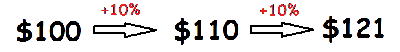Math Calculators, Lessons and Formulas

It is time to solve your math problem

mathportal.org

# Compound interest calculator

Compound Interest is calculated on the initial payment and also on the interest of previous periods.

Example: Suppose you give \$100 to a bank which pays you 10% compound interest at the end of every year. After one year you will have \$100 + 10% = \$110, and after two years you will have \$110 + 10% = \$121.Problem If you deposit$3500 into an account paying 10% annual interest compounded monthly , how much money will be in the account after 8 years?

Result:

The amount is $7763.37 and the interest is$4263.37.

Explanation

STEP 1: To find amount we use formula:

 $$A = P \left( 1 + \frac{r}{n} \right)^{\Large{n \cdot t}}$$ A = total amount P = principal or amount of money deposited, r = annual interest rate n = number of times compounded per year t = time in years

In this example we have

$$P = \3500 ~,~ r = 10 \% ~ , ~ n = 12 ~ \text{and} ~ t = 8 ~ \text{years}$$

After plugging the given information we have

\begin{aligned} A &= 3500 \left( 1 + \frac{ 0.1 }{ 12 } \right)^{\Large{ 12 \cdot 8 }} \\ A &= 3500 \cdot { 1.008333 } ^ { 96 } \\ A &= 3500 \cdot 2.218105 \\ A &= 7763.37 \end{aligned}

STEP 2: To find interest we use formula $A = P + I$, since $A =$7763.37 $and$ P = $3500$ we have:

\begin{aligned} A &= P + I \\ 7763.37 &= 3500 + I \\ I &= 7763.37 - 3500 \\ I &= 4263.37 \end{aligned}

## Report an Error !

Script name : compound-interest-calculator

Imputed values: 0 , 3500 , 10 , 8 , 1 , 4

Comment (optional)

Email (optional)

## Share Result

Compound Interest Calculator
0 1 2 3 4 5 6 7 8 9 . del
Show me the solution without an explanation
Compound Interest Calculator
0 1 2 3 4 5 6 7 8 9 . del
Show me the solution without an explanation
examples
example 1:
What will a deposit of $\color{blue}{\$4500}$at$\color{blue}{7\%}$compounded$\color{blue}{\text{yearly}}$interest be worth if left in the bank for$\color{blue}{\text{9 years}}$? example 2: What will a deposit of$\color{blue}{\$3500}$ at $\color{blue}{10\,\%}$ compounded $\color{blue}{\text{monthly}}$ be worth if left in the bank for $\color{blue}{8 \, \text{years}}$ ?
example 3:
How much money would you need to deposit today at $\color{blue}{8\% \, \text{annual}}$ interest compounded $\color{blue}{\text{monthly}}$ to have $\color{blue}{\$1200}$in the account after$\color{blue}{12 \, \text{years}} \, \text{?}$example 4: Find the present value of$\color{blue}{\$1000}$ to be received at the end of $\color{blue}{2 \, \text{years}}$ at a $\color{blue}{12\%}$ nominal annual interest rate compounded $\color{blue}{\text{quarterly}}$.
example 5:
What annual interest rate is implied if you lend someone $\color{blue}{\$1700}$and are repaid$\color{blue}{\$1910}$ in $\color{blue}{\text{two years}}$?
example 6:
Suppose that a savings account is compounded $\color{blue}{\text{monthly}}$ with a principal of $\color{blue}{\$1350}$. After$\color{blue}{8 \, \text{months}}$, the amount increased to$\color{blue}{\$1424}$. What was the per annum interest rate?
example 7:
How long does it take for $\color{blue}{\$4300}$to grow into$\color{blue}{\$6720}$ at $\color{blue}{9\,\%}$ compounded $\color{blue}{\text{quarterly}}$?

Quick Calculator Search